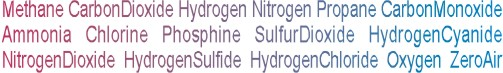Calibration gas blends, mixes and accessories
NON-REACTIVE SINGLE GAS > HYDROGEN :    17 Items found.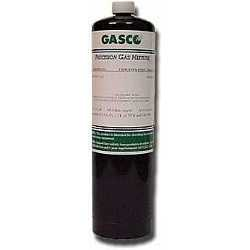(1206)

## NORLAB Equivalent  P104920

Norlab Equivalent Calibration Gas 20% LEL Hydrogen Balance air 17L Liter cylinder (Made by Gasco)

Configure

\$32.00(1207)

## NORLAB Equivalent  P104925

Norlab Equivalent Calibration Gas 25% LEL Hydrogen Balance air 17L Liter cylinder (Made by Gasco)

Configure

\$32.00(1208)

## NORLAB Equivalent  P104940

Norlab Equivalent Calibration Gas 40% LEL Hydrogen Balance air 17L Liter cylinder (Made by Gasco)

Configure

\$32.00(1209)

## NORLAB Equivalent  P104950

Norlab Equivalent Calibration Gas 50% LEL Hydrogen Balance air 17L Liter cylinder (Made by Gasco)

Configure

\$32.00(1210)

## NORLAB Equivalent  P104950N

Norlab Equivalent Calibration Gas 2.0% vol. Hydrogen Balance nitrogen 17L Liter cylinder (Made by Gasco)

Configure

\$32.00(1205)

## NORLAB Equivalent  P1049

Norlab Equivalent Calibration Gas 100% vol. Hydrogen Balance - 17L Liter cylinder (Made by Gasco)

Configure

\$32.00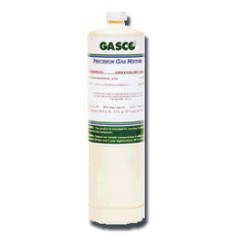(1214)

## NORLAB Equivalent  HP104950

Norlab Equivalent Calibration Gas 50% LEL Hydrogen Balance air 34LS Liter cylinder (Made by Gasco)

Configure

\$65.00(1213)

## NORLAB Equivalent  HP104925

Norlab Equivalent Calibration Gas 25% LEL Hydrogen Balance air 34LS Liter cylinder (Made by Gasco)

Configure

\$65.00(1215)

## NORLAB Equivalent  HP104950N

Norlab Equivalent Calibration Gas 2.0% vol. Hydrogen Balance nitrogen 34LS Liter cylinder (Made by Gasco)

Configure

\$65.00(1212)

## NORLAB Equivalent  HP104920

Norlab Equivalent Calibration Gas 20% LEL Hydrogen Balance air 34LS Liter cylinder (Made by Gasco)

Configure

\$65.00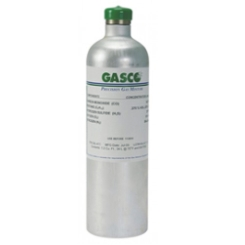(1211)

## NORLAB Equivalent  HP1049

Norlab Equivalent Calibration Gas 100% vol. Hydrogen Balance - 34L Liter cylinder (Made by Gasco)

Configure

\$81.00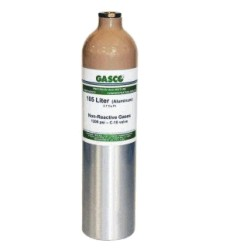(1221)

## NORLAB Equivalent  10491000

Norlab Equivalent Calibration Gas 1000 ppm Hydrogen Balance air 105L Liter cylinder (Made by Gasco)

Configure

\$97.20(1216)

## NORLAB Equivalent  104920

Norlab Equivalent Calibration Gas 20% LEL Hydrogen Balance air 105L Liter cylinder (Made by Gasco)

Configure

\$97.20(1217)

## NORLAB Equivalent  104925

Norlab Equivalent Calibration Gas 25% LEL Hydrogen Balance air 105L Liter cylinder (Made by Gasco)

Configure

\$97.20(1218)

## NORLAB Equivalent  104950

Norlab Equivalent Calibration Gas 50% LEL Hydrogen Balance air 105L Liter cylinder (Made by Gasco)

Configure

\$97.20(1219)

## NORLAB Equivalent  104950N

Norlab Equivalent Calibration Gas 2.0% vol. Hydrogen Balance nitrogen 105L Liter cylinder (Made by Gasco)

Configure

\$97.20(1220)

## NORLAB Equivalent  1049500

Norlab Equivalent Calibration Gas 500 ppm Hydrogen Balance air 105L Liter cylinder (Made by Gasco)

Configure

\$97.20